#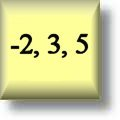Integers Worksheets

## Dynamically Created Integers Worksheets

Here is a graphic preview for all of the Integers Worksheets. You can select different variables to customize these Integers Worksheets for your needs. The Integers Worksheets are randomly created and will never repeat so you have an endless supply of quality Integers Worksheets to use in the classroom or at home. Our Integers Worksheets are free to download, easy to use, and very flexible.

## Quick Link for All Integers Worksheets

Click the image to be taken to that Integers Worksheet.

##### Representation ofIntegers Worksheets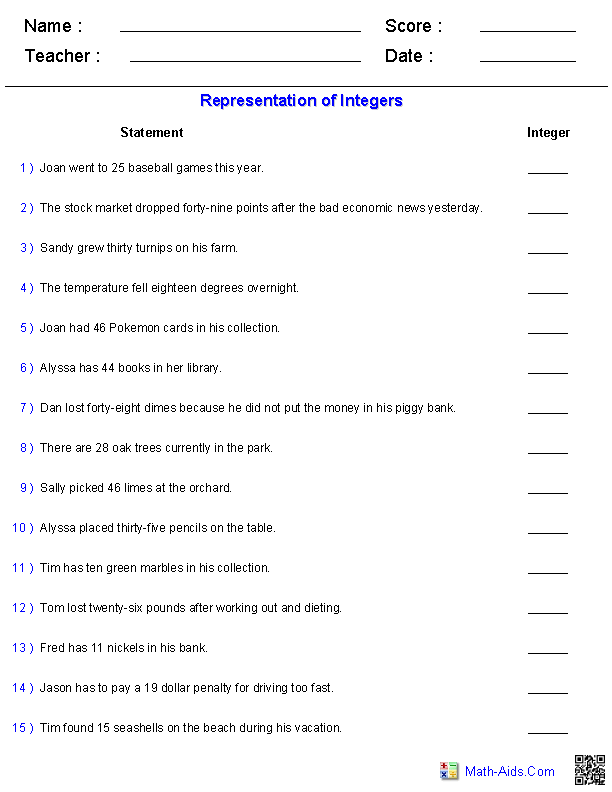##### Absolute Value ofIntegers Worksheets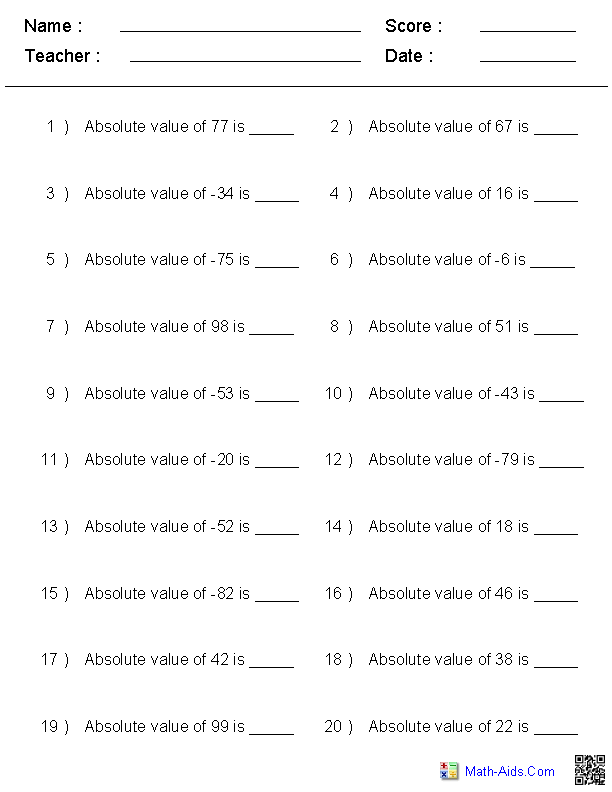##### Opposite Value ofIntegers Worksheets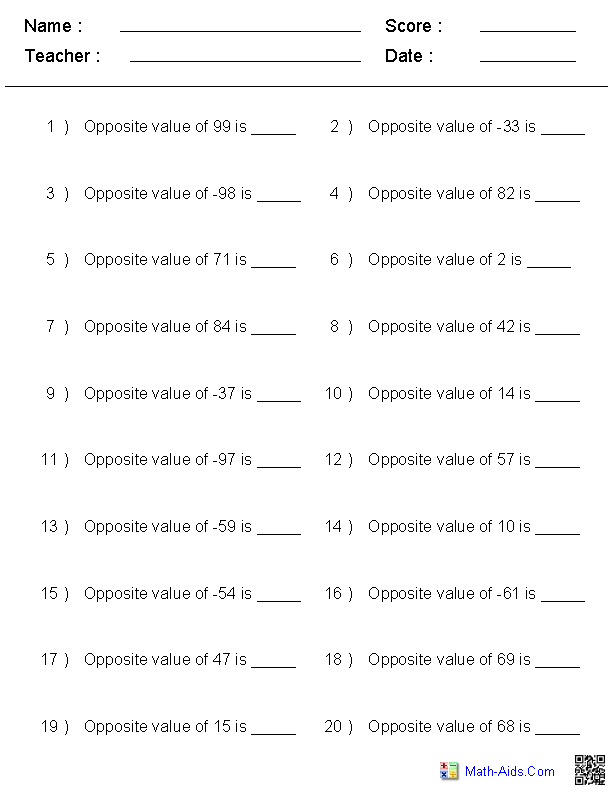##### One Less & One MoreIntegers Worksheets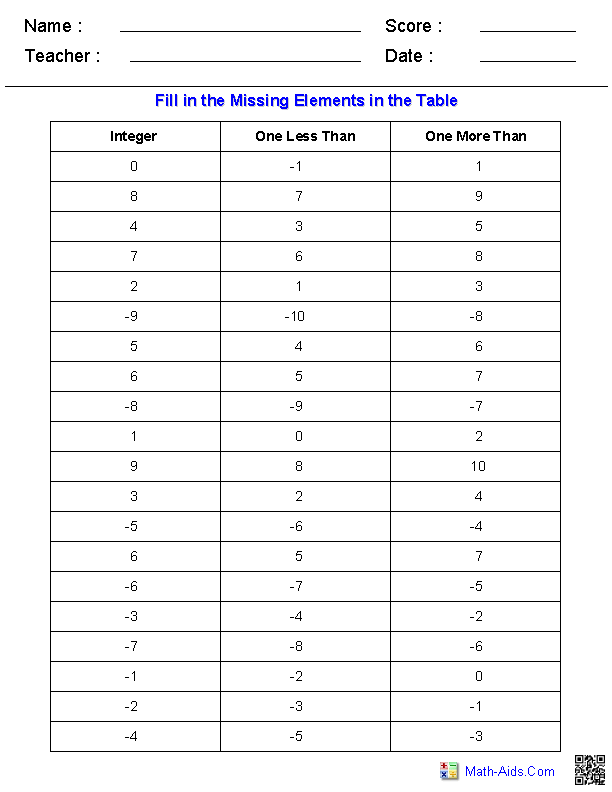##### ComparingIntegers Worksheets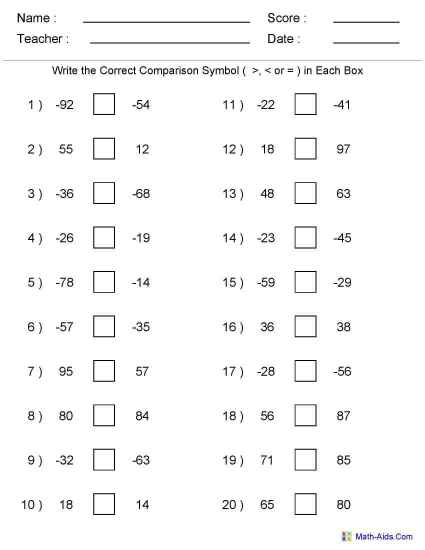##### Greatest / SmallestIntegers Worksheets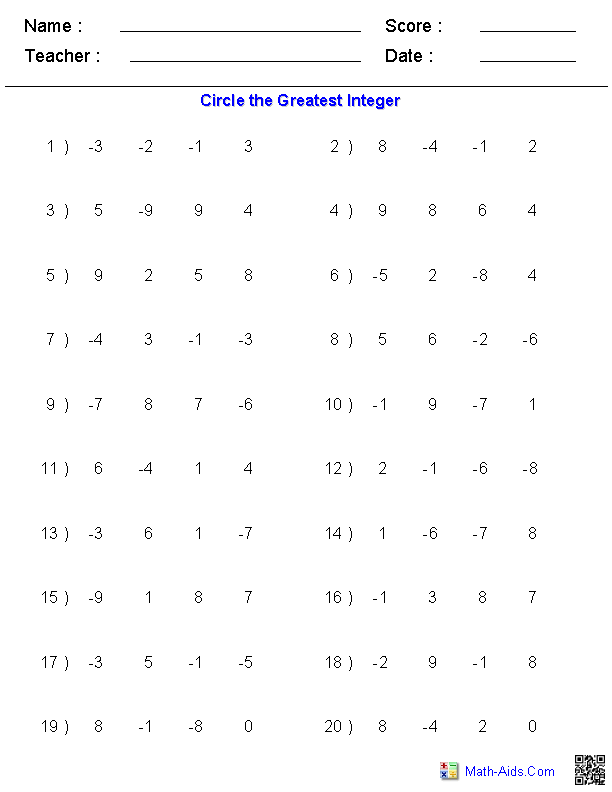##### Arranging  Orders ofIntegers Worksheets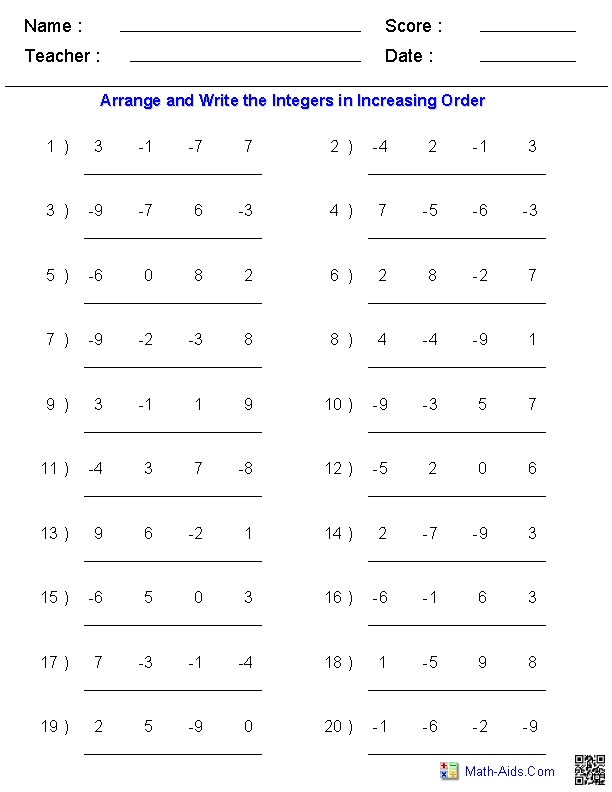##### OrderingWhole Numbers Worksheets##### 1 or 2 Digit Addition - 2 TermsIntegers Worksheets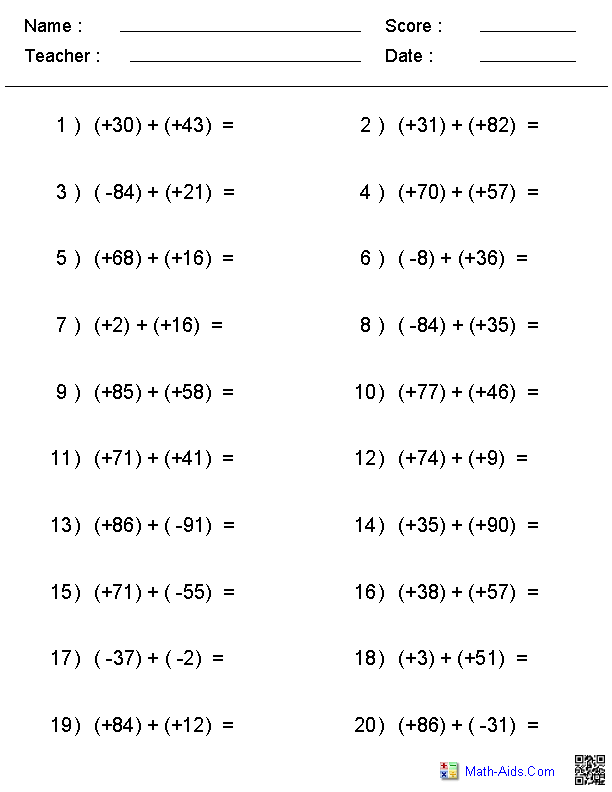##### 1 or 2 Digit Addition - 3 TermsIntegers Worksheets##### 1 or 2 Digit Addition - 4 TermsIntegers Worksheets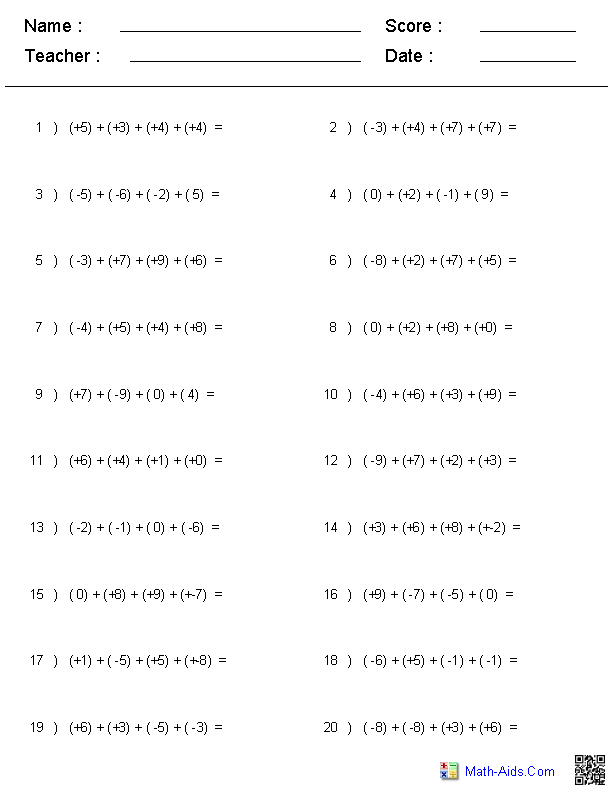##### 1 or 2 Digit SubtractionIntegers Worksheets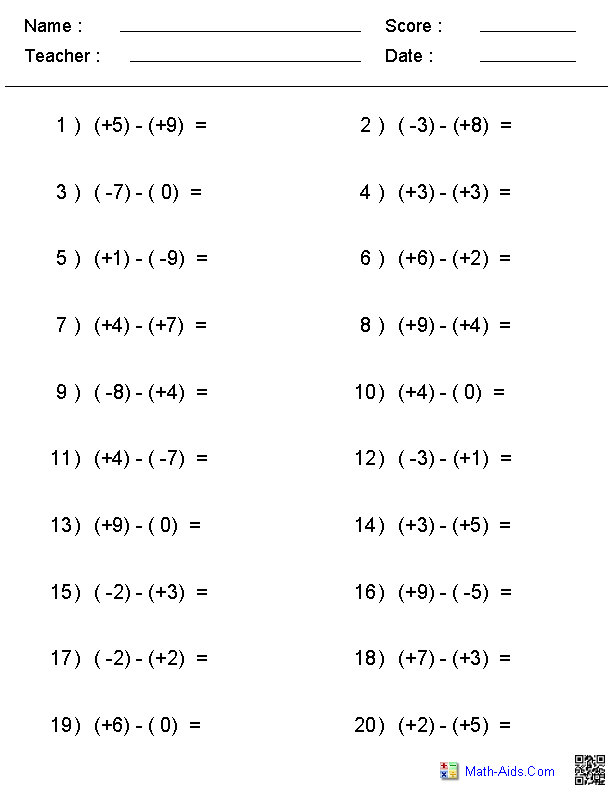##### 1 or 2 Digit MultiplicationIntegers Worksheets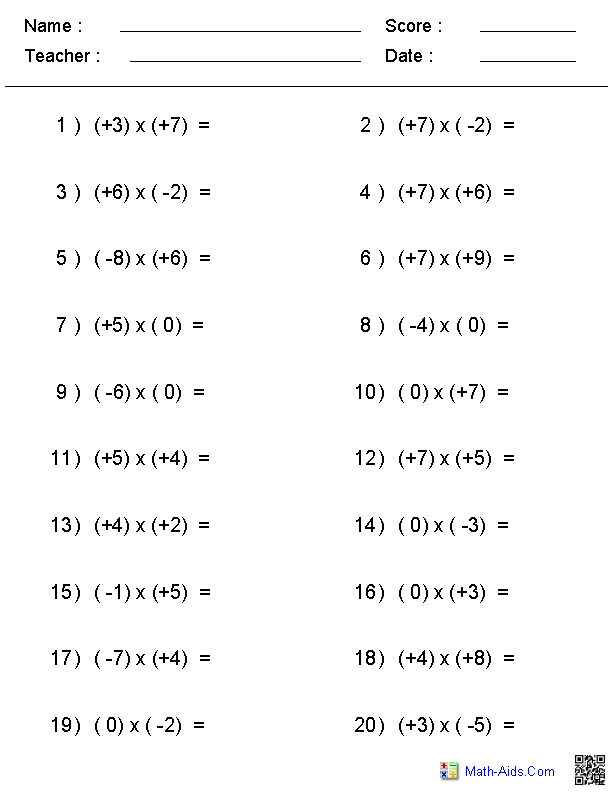##### 1 or 2 Digit DivisionIntegers Worksheets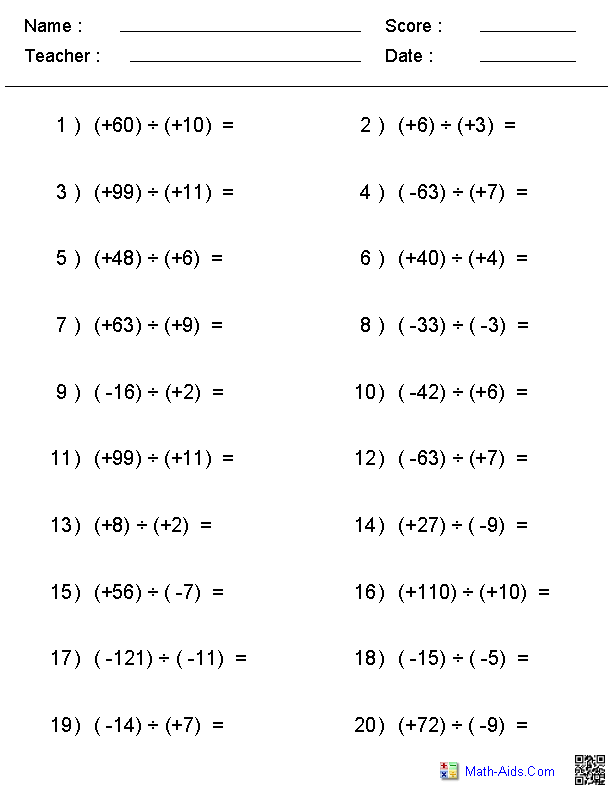##### 1 or 2 Digit Mixed ProblemsIntegers Worksheets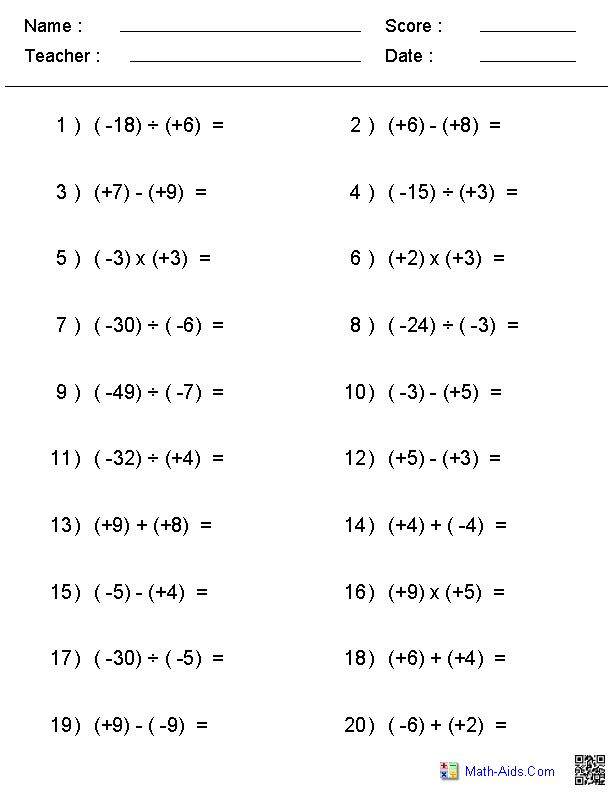Recommended Videos

## Detailed Description for All Integers Worksheets

Representation of Integers Worksheets
These integers worksheets will produce word problems for the students to identify the integer represented in the statement.

Absolute Value of Integers Worksheets
These integers worksheets may be configured for either 1 or 2 digit problems about absolute value of integers.

Opposite Value of Integers Worksheets
These integers worksheets may be configured for either 1 or 2 digit problems about opposite value of integers.

One Less & One More Integers Worksheets
These integers worksheets will produce problems where the student will fill in the table with one less and one more of the integer listed.

Comparing Integers Worksheets
These integers worksheets will dynamically produce problems based on your selections. You may select 1 though 6 digits problems, use numbers in the range of 1 through 20, or randomly generate problems with mixed digits based on your selection. You may select positive, negative or mixed sign problems.

Greatest / Smallest Integers Worksheets
These integers worksheets will produce problems with 4 diffent integers and the student will circle the greatest or smallest integer.

Arranging Orders of Integers Worksheets
These integers worksheets will produce problems with 4 diffent integers and the student will arrange the integers either in increasing or decreasing order.

Ordering Whole Numbers Worksheets
These integers worksheet will produce problems for ordering 4 whole numbers. You may select the four numbers to have the same number of digits, or produce four whole numbers with different numbers of digits. You may select between 3 and 6 digits for the problems. You may select the ordering of the problems from greatest to least, least to greatest, or both.

1 or 2 Digit Addition - 2 Terms Integers Worksheets
These integers worksheets may be configured for either 1 or 2 digit horizontal addition problems with 2 terms. The terms may be selected to be positive, negative or mixed sign.

1 or 2 Digit Addition - 3 Terms Integers Worksheets
These integers worksheets may be configured for either 1 or 2 digit horizontal addition problems with 3 terms. The terms may be selected to be positive, negative or mixed sign.

1 or 2 Digit Addition - 4 Terms Integers Worksheets
These integers worksheets may be configured for either 1 or 2 digit horizontal addition problems with 4 terms. The terms may be selected to be positive, negative or mixed sign.

1 or 2 Digit Subtraction Integers Worksheets
These integers worksheets may be configured for either single or multiple digit horizontal subtraction problems with the numbers being positive, negative or mixed signs.

1 or 2 Digit Multiplication Integers Worksheets
These integers worksheets may be configured for either single or multiple digit horizontal multiplication problems with the numbers being positive, negative or mixed signs.

1 or 2 Digit Division Integers Worksheets
These integers worksheets may be configured for either single or multiple digit horizontal division problems with the numbers being positive, negative or mixed signs.

1 or 2 Digit Mixed Problems Integers Worksheets
These integers worksheets may be configured for either single or multiple digit horizontal mixed problems for addition, subtraction, multiplication and division.